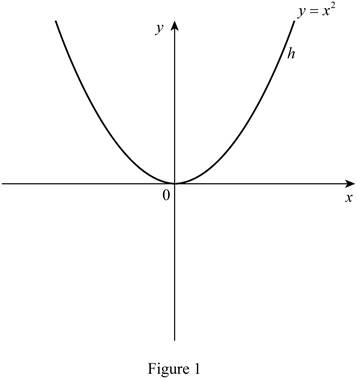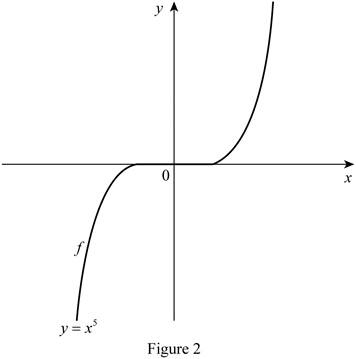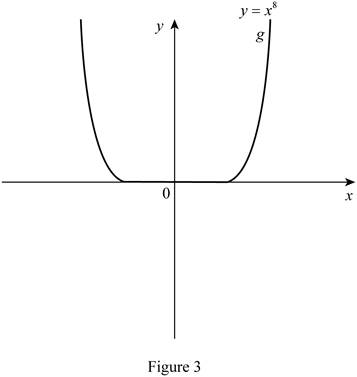# To match: The equation y = x 2 with its graph without using a computer or graphing calculator.### Single Variable Calculus: Concepts...

4th Edition
James Stewart
Publisher: Cengage Learning
ISBN: 9781337687805### Single Variable Calculus: Concepts...

4th Edition
James Stewart
Publisher: Cengage Learning
ISBN: 9781337687805

#### Solutions

Chapter 1.2, Problem 3E

(a)

To determine

## To match: The equation y=x2 with its graph without using a computer or graphing calculator.

Expert Solution

The equation y=x2 is match with the graph h.

### Explanation of Solution

The equation y=x2 represents a polynomial function that is quadratic in nature. For the null value of x , the value of y is zero, so the graph of the equation passes through the origin (0,0) and symmetric about y-axis. Thus, the graph of y=x2 is shown below in Figure 1.From Figure 1, it can be concluded that the equation y=x2 matches with the graph h.

(b)

To determine

### To match: The equation y=x5 with its graph without using a computer or graphing calculator.

Expert Solution

The equation y=x5 is match with the graph f.

### Explanation of Solution

The equation y=x5 represents an odd polynomial function of degree 5. For the null value of x , the value of y is zero, so the graph of the equation passes through the origin (0,0) . Since the function is odd, it is symmetric about the origin. Thus, the graph of an odd polynomial function of degree 5 is shown below in Figure 2.From Figure 2, it can be concluded that the equation y=x5 matches with the graph f.

(c)

To determine

### To match: The equation y=x8 with its graph without using a computer or graphing calculator.

Expert Solution

Solution:

The equation y=x8 is match with the graph g.

### Explanation of Solution

The equation y=x8 represents an even polynomial function. For the null value of x , the value of y is zero, so the graph of the equation passes through the origin (0,0) . Since the function is even, it is symmetric about y-axis. Thus, the graph of an even polynomial function of degree 8 is shown below in Figure 3.From Figure 3, it can be concluded that the equation y=x8 matches with the graph g.

### Have a homework question?

Subscribe to bartleby learn! Ask subject matter experts 30 homework questions each month. Plus, you’ll have access to millions of step-by-step textbook answers!